Courses
Courses for Kids
Free study material
Free LIVE classes
More

# International Place Value Chart## Introduction to Number System

In mathematics, you read any number by grouping them in the threes in a big number, and you all learn this system in the place value map. So, in the place value map, the integers are grouped in the threes in a big number, and the number is read from left to right as billion, million, or thousands. You’ll learn further about the place value map below.

Can you read the number 12345?Numbers

Is it one, two, three, four, and five...NO

Now read this 12,345. Is it twelve thousand three hundred and forty-five? YES!

## Place Value Chart

A place value chart is a table used to determine the value of each digit in a number on its numerical position. For example, in the number 92,735, the place value of:

• 5 is Ones

• 3 is Tens

• 7 is Hundreds

• 2 is Thousands

• 9 is ten thousand.

The diagram below illustrates the above example in more detail.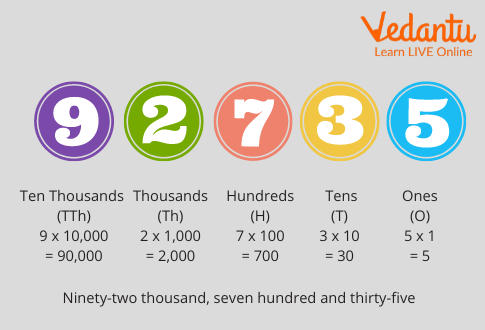Place Value of the Number

In a number, the same digit might have several values depending on its position. For illustration, 4 in 524 denotes the one place, whereas it denotes the knockouts place in 948.

A universal map is used to ensure that the integers of a number are aligned rightly according to their positional values. To make the process easier, larger figures are broken into smaller groups, separated by commas, similar to ones, tens, hundreds, and so on.

## Indian Place Value Chart

The Indian place value chart or map is a table that uses the Indian numeric system to determine the value of each digit in a number based on its position. Figures are employed in mathematics for counting, measures, and comparisons. Each digit in a number has its value grounded on where it falls on the place value map. A number's place value indicates how much a digit is worth in relation to its position. We divide the figures into groups with the help of a comma and a division to minimize confusion while reading particularly large figures.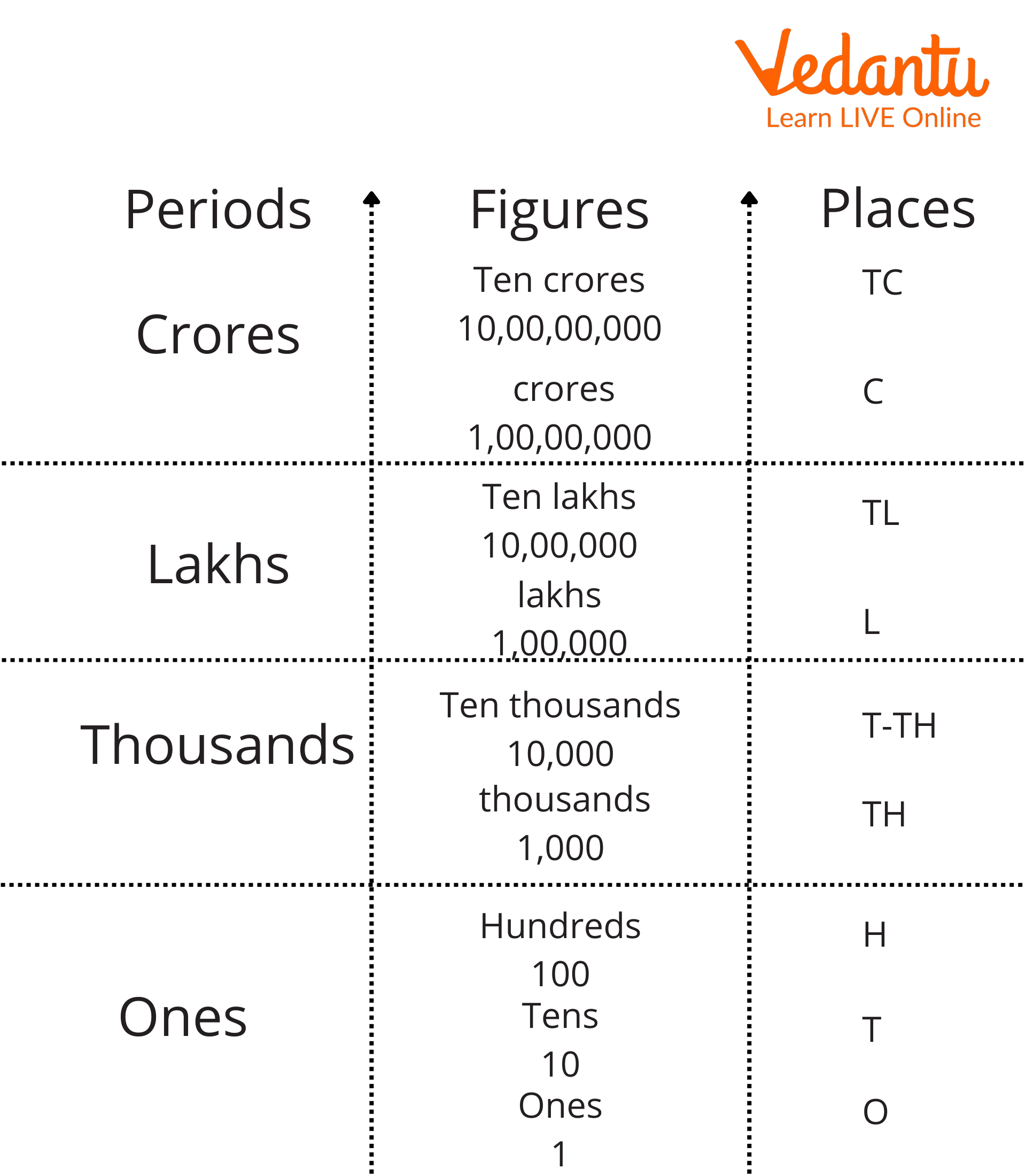Indian Place Value Chart

## International Place Value Chart

For the nine places from right to left in the International place-value system, there are three periods: ones, thousands, and millions. Three place values make up one's period. Hundreds, tens, and thousands. The thousands after that are made up of one, ten, and hundred-thousands. The trend continues, and the next three spots all make millions. As a result, when writing a number in the international place-value system, we place commas after every three digits from the right.

To read the number, we read all the digits in the same period, then the period's name from left to right.

According to the international numeral system, the chart below aids us in determining and comparing the place value of digits in numbers up to millions.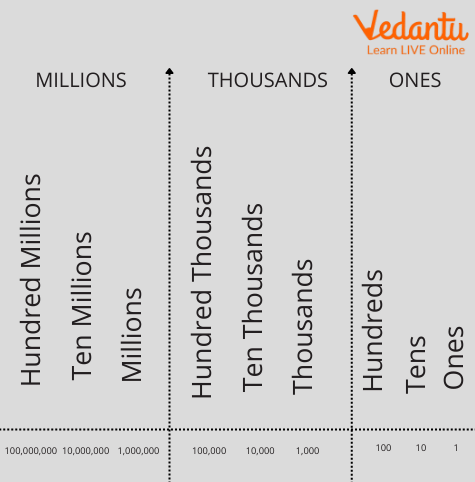International Place Value Chart

## Solved Examples

1. Write the place value of 4 and 7 in 72,425.

Solution: The place value of 4 is in the hundreds place. So its place value is 400.
The place value of 7 is at the ten thousand’s place. So its place value is 70,000.

1. Write the place value of 5 and 3 in 57,324,208.

Solution: The place value of 5 is at ten million places. So its place value is 50,000,000

The place value of 3 is at the hundred thousand places. So its place value is 300,000.

1. Write the number 2319617 in the Indian place value chart and the International place value chart. Identify the numbers having the same place values in both charts.

Solution: Firstly, we will write the given number in both place value charts.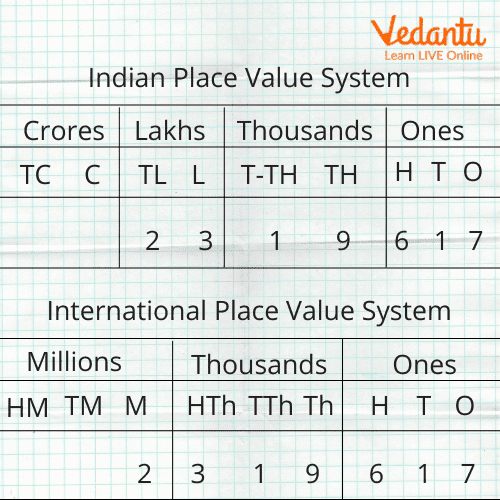Place Value Chart

In the Indian place value chart, the number 2319617 is divided into three periods: ones, thousands, and lakhs, and in the International place value chart, it is ones, thousands, and millions.

Starting from the right, the numbers 7, 1, 6, 9, and 1 have the same place values, as seen in the place value charts.

1. What is the place value of 9 in 3921919 in international place value number systems?

Solution: Firstly, we will write the given number in the International place value chart.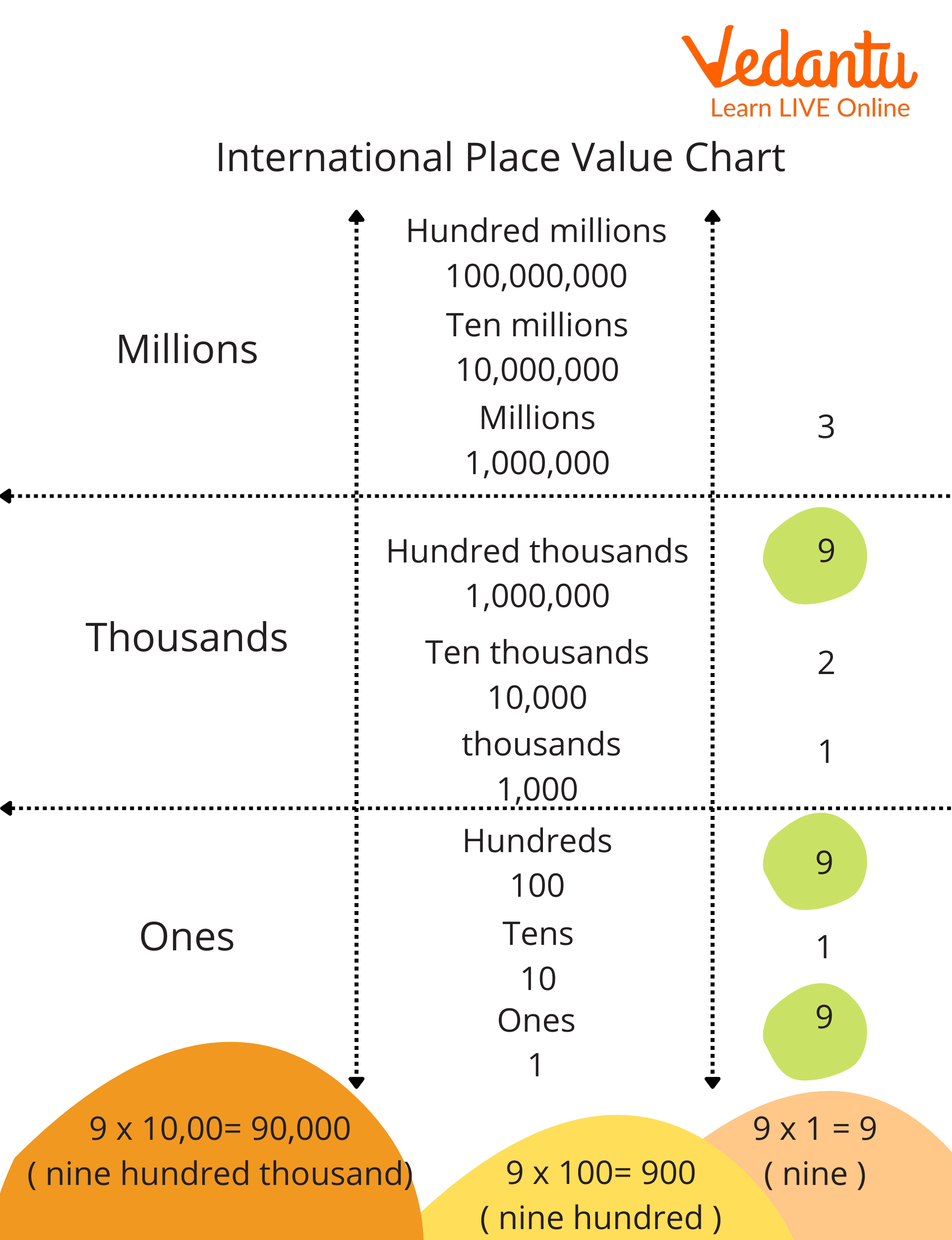International Place Value Chart of 3921919

As we start from the right, we get the place value of the first 9 is 9, the second 9 is 900 and the third 9 is 900,000.

Therefore, the place value of the first 9 is 9, the second 9 is 900 and the third 9 is 900,000.

1. What is the place value of 5 in 3521519 in international place value number systems?

Ans. Hundred Thousand and Hundred

2. What is the place value of 6 in 561549 in Indian place value number systems?

Ans. Ten thousand

## Conclusion

In this article, a place value chart was introduced with an example of numbers. A place value chart is used to determine the value of each digit in a number. In mathematics, we have two types of place value charts one is an Indian place value chart, and another is an international place value chart. In the Indian place value chart, lakhs and crores are followed after thousand, but in international, millions and billions are used, respectively.

Last updated date: 01st Oct 2023
Total views: 123.3k
Views today: 4.23k

## FAQs on International Place Value Chart

1. What is the difference between the Indian place value chart and the international place value chart?

The International place value chart divides nine places into three periods: ones, thousands, and millions, followed by billions, and so on. Ones, tens, hundreds, thousands, ten thousand, lakhs, ten lakhs, crores, and ten crores are all used in the Indian place value chart.

2. What is a place value chart?

A universal chart or map is used to ensure that the integers of a number are aligned rightly according to their positional values. To make the process easier, larger figures are broken and separated by commas, similar to ones, tens, hundreds, and so on. This is called a place value map.